## Measurement of Information in Communication System:

Having said what information is not (it is not meaning), we now state specifically what information is. Accordingly, information is defined as the choice of one message out of a finite set of messages. Meaning is immaterial, in this sense, a table of random numbers may well contain as much Measurement of Information in Communication System as a table of world track-and-field records. Indeed, it may well be that a cheap fiction book contains more information than this textbook, if it happens to contain a larger number of choices from a set of possible messages (the set being the complete English language in this case). Also, when Measurement of Information in Communication System, it must be taken into account that some choices are more likely than others and therefore contain less information. Any choice that has a probability of 1, ,i.e., is completely unavoidable, is fully redundant and, therefore, contains no information. An example is the letter “u” in English when it follows the letter “q.”

### The Binary System:

This system can be illustrated in its simplest form as a series of lights and switches. Each condition is represented by a one or a zero (see Figure 13-1).Each light represents a numerical weight (bit) as indicated. This group represents a 5-bit system which, if all the switches were in the off position, would equal 0 (zero). The total decimal number represented by the four-switch light combinations is equal to the decimal number 31 (the sum of the bit weights). This method of on/off can be represented by voltage levels, with a 1 equal to 5 V and a 0 equal to 0 V. This method provides a sharp (high) signal-to-noise ratio (noise usually being measured in millivolt levels) and helps maintain accurate data transmission. The simplicity, speed, and accuracy of this system give it many advantages over its analog counterpart.

### Coding:

In Measurement of Information in Communication System, we have so far concentrated on a choice of one from T equiprobable events, using the binary system, thus the number of bits involved has always been an integer. In fact, if we do use the binary system for signaling, the number of bits required will always be an integer. For example, it is not possible to choose one from a set of 13 equiprobable events in the binary system by giving 3.7 bits (log2 13 = 3.7). It is necessary to give 4 bits of information, which corresponds to having the switching system of Figure 13-1 with the last three places never used. The efficiency of using a binary system for the selection of one of 13 equiprobable events is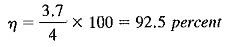which is considered a high efficiency. The situation is that a choice of one from 13 conveys 3.7 bits of information, but if we are going to use a binary system of selection or signaling, 4 bits must be given and the resulting inefficiency accepted.

At this point, it is worth noting that the binary system is used widely but not exclusively. The decimal system is also used, and here the unit of information is the decimal digit, or dit. A choice of one from a set of 10 equiprobable events involves 1 dit of information and may be made, in the decimal system, with a rotary switch. It is simple to calculate that since we have log2 10 = 3.32,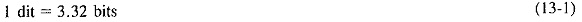Just as a matter of interest, it is possible to compare the efficiency of the two systems by noting that log10 13 = 1.11, and thus the choice of one Out of 13 equiprobable events involves 1.11 dits. Following the reasoning of Figure 13-1, 100 switching positions must be provided in the decimal switching system, so that 2 dits of information will be given to indicate the choice. Efficiency is thus η = 1.11/2 X 100 = 55.5 percent, decidedly lower than in the binary system. Although this is only an isolated instance, it is still true to say that in general a binary switching or coding system is more efficient than a decimal system.

### Baudot Code:

If words are to be sent by a communications system, some form of coding must be used. If the total number of words or ideas is relatively small, a different symbol may be used for each word or object. The Egyptians did this for words with hieroglyphs, or picture writing, and we do it for objects with circuit symbols. However, since the English language contains at least 800,000 words and is still growing, this method is out of the question. Alternatively, a different pulse, perhaps having a different width or amplitude, may be used for each letter and symbol. Since there are 26 letters in English and roughly the same number of other symbols, this gives a total of about 50 different pulses. Such a system could be used, but it never is, because it would be very vulnerable to distortion by noise.

If we consider pulse-amplitude variation and amplitude modulation, then each symbol in such a system would differ by 2 percent of modulation from the previous, this being only one-fiftieth of the total amplitude range. Thus the word “stop” might be transmitted as /38/40/30/32/, each figure being the appropriate percentage modulation. Suppose a very small noise pulse, having an amplitude of only one-fiftieth of the peak modulation amplitude, happens to superimpose itself on the transmitted signal at that instant. This signal will be transformed into /40/42/32/34/, which reads “tupq” in this system and is quite meaningless. It is obvious that a better system must be found. As a result of this, almost all the systems in use are binary systems, in which the sending device sends fully modulated pulses (“marks”) or no-pulses (“spaces”). Noise now has to compete with the full power of the transmitter, and it will be a very large noise pulse indeed that will convert a transmitted mark into a space, or vice versa.

Since Measurement of Information in Communication System in English is drawn from 26 choices (letters), there must be on the average more than 1 bit per letter. In fact, since log2 26 = 4.7 and a binary sending system is to be used, each letter must be represented by 5 bits. If all symbols are included, the total number of different signals nears 60. The system is in use with tele-typewriters, whose keyboards are similar to those of ordinary typewriters. It is thus convenient to retain 5 bits per symbol and to have carriage-shift signals for chang­ing over from letters to numerals, or vice versa.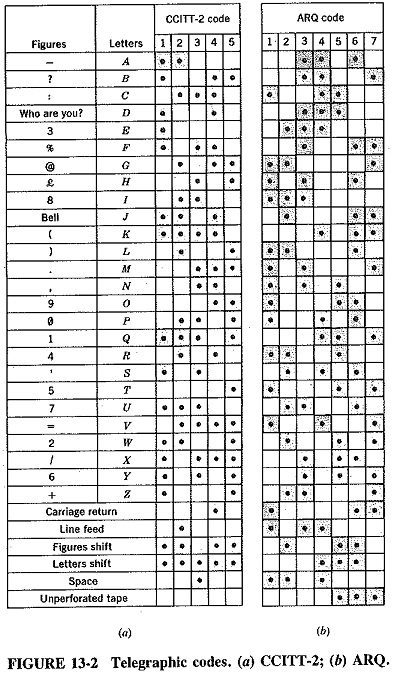The CCITT No. 2 code shown in Figure 13-2a is an example of how a series of five binary signals can indicate any one from up to 60 letters and other symbols. The code is based on an earlier one proposed by J. M. E. Baudot, the only difference being an altered allocation of code symbols to various letters. In the middle of a message, a word of n letters is indicated by n + 1 bits; the last bit is used for the space as shown in Figure 13-3.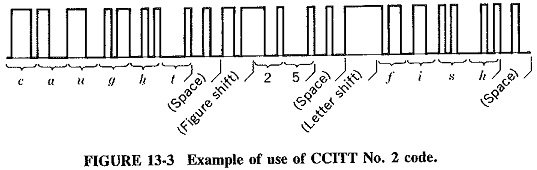A telegraphic code known as the ARQ code (automatic request for repetition) was developed from the Baudot code by H. C. A. Van Duuren in the late 1940s, and is an example of an error-detecting code widely used in radio telegraphy. As shown, 7 bits are used for each symbol, but of the 128 possible combinations that exist, only those containing 3 marks and 4 spaces are used. There are 35 of these, and 32 of them are used as shown in Figure 13-2b. The advantage of this system is that it offers protection against single errors. If a signal arrives so mutilated that some of the code groups contain a mark-to-space proportion other than 3:4, an ARQ signal is sent, and the mutilated information is re-transmitted. There is no such provision for the detection of errors in the Baudot-based codes, but they do have the advantage of requiring only 5 bits per symbol, as opposed to 7 here.

### The Hartley law:

The Baudot code was shown as an example of a simple and widely used binary code, but it may also be employed as a vehicle for providing a very fundamental and important law of Measurement of Information in Communication System theory. This is the Hartley law and may be demonstrated by logic.

A quick glance at the CCITT-2 code of Figure 13-2a reveals that, on the average, just as many bits of information are indicated by pulses as by no-pulses. This means of course, that the signaling rate in pulses per second depends on the informa­tion rate in bits per second at that instant. Now the pulse rate is by no means constant. If the letters “Y” and “R” are sent one after the other, the pulse rate will be at its maximum and exactly equal to half the bit rate. At the other end of the scale, the letter “E,” followed by “T,” would provide a period of time during which no pulses are sent. Accordingly, it is seen that when information is sent in a binary code at a rate of b bits per second, the instantaneous pulse rate varies randomly between b/2 pulses per second and zero. It follows that a band of frequencies, rather than just a single frequency, is required to transmit information at a certain rate with a particular system. It will be recalled from earlier topics that pulses consist of the fundamental frequency and harmonics, in certain proportions. However, if the harmonics are filtered out at the source, and only fundamentals are sent, the original pulses can be re-created at the destination (with multivibrators). This being the case, the highest frequency required to pass b bits per second in this system is b/2 Hz (the lowest frequency is still 0). It may thus be said that, if a binary coding system is used, the channel capacity in bits per second is equal to twice the bandwidth in hertz. This is a special case of the Hartley law. The general case states that, in the total absence of noise,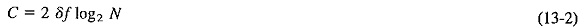where

C = channel capacity, bits per second

δf = channel bandwidth, Hz

N = number of coding levels

When the binary coding system is used, the above general case is reduced to C = 2δf, since log2 2 = 1. The Hartley law shows that the bandwidth required to transmit information at a given rate is proportional to the information rate. Also, in the absence of noise, the Hartley law shows that the greater the number of levels in the coding system, the greater the information rate that may be sent through a channel. What happens when noise is present was indicated in the preceding section, (i.e.,. “tupq” for “stop”). Meanwhile, extending the Hartley law to its logical conclusion, as was done by the originator, we have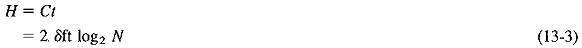where

H = total information sent in a time t, bits

t = time, seconds.

The foregoing assumes, of course, that an information source of sufficient capacity is connected to the channel.

Scroll to Top